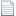# 广义指数均值的Schur凸性和Schur几何凹性

Journal of Mathematical Inequalities，Volume 3, Number 2 (2009), 217–225.

SCHUR CONVEXITY AND SCHUR–GEOMETRICALLY CONCAVITY OF GENERALIZED EXPONENT MEAN

DA-MAO LI AND HUAN-NAN SHI

Abstract. The monotonicity, the Schur-convexity and the Schur-geometrically convexity with
variables $(x,y) \in R^2_{++}$ for fixed a of the generalized exponent mean $I_a(x,y)$ is proved. Besides, the monotonicity with parameters a in $R$ for fixed $(x,y)$ of $I_a(x,y)$ is discussed by

using the hyperbolic composite function. Furthermore, some new inequalities are obtained.

Mathematics subject classification (2000): 26D15, 26A51.

Keywords and phrases: generalized exponent mean, monotonicity, Schur-convexity, Schur geometrically concavity inequality, hyperbolic function.SCHUR CONVEXITY AND SCHUR–GEOMETRICALLY CONCAVITY OF GENERALIZED EXPONENT MEAN.pdf

https://blog.sciencenet.cn/blog-3453835-1273179.html

## 全部精选博文导读

GMT+8, 2022-6-30 17:27